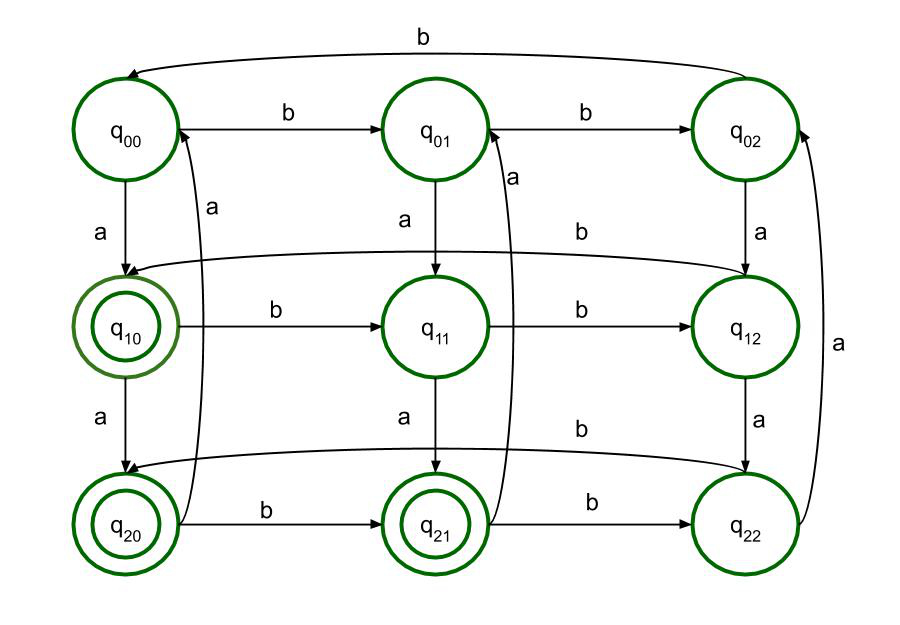Open in App
Not now

# DFA of Regular Language L ={w ∈ {a,b}* : Na(w) mod 3 > Nb(w) mod 3}

• Difficulty Level : Hard
• Last Updated : 16 Oct, 2020

In this article, we will design the Deterministic Finite Automata of the Regular Language L ={w ∈ {a, b}* : Na(w) mod 3 > Nb(w) mod 3}.

Regular Expression can be anything from a terminal symbol, ∅, to union of two regular expressions (R1 + R2 ), their intersection (R1 + R2) or the regular expression’s closure (R1*) or a ∈ Σ, where Σ is the finite set of input symbols, which is also a regular expression of the language {a}.

Regular Language is a language which can be expressed in terms of regular expression.

Examples of Regular Expressions :

1. Regular expression of set of all strings Σ = {a, b} with exactly one a.
`b*ab* `
2. Regular expression of set of all strings Σ = {a, b} starting with prefix ab.
`ab(a+b)* `

Problem :
Regular Language L = {w ∈ {a, b}* : Na(w) mod 3 > Nb(w) mod 3} means the language accepts all the strings where the count of a’s in string modulus 3 is greater than the count of b’s modulus 3.

Examples :

```Input : aaabbbb

Output : Not Accepted

Reason : Na(w) = 3; 3 mod 3 = 0 and Nb(w) = 4; 4 mod 3 = 1. So Na(w) mod 3 !> Nb(w) mod 3

Input : aabbbb

Output : Accepted

Reason : Na(w) = 2 and Nb(w) = 4; 2 mod 3 = 2 and 4 mod 3 = 1. So Na(w) mod 3 > Nb(w) mod 3
```

Approach :
Since it is modulus 3, the remainders can be 0, 1, 2.
When Na(w) mod 3 = 0, then whatever be the value of Nb(w) mod 3, the language will not be accepted.
When Na(w) mod 3 = 1, then the language is accepted when Nb(w) mod 3 = 0.
Again, when Na(w) mod 3 = 2, then the language is accepted when Nb(w) mod 3 = 0 or 1.
This can be explained in a tabular form:

0

0

NOT ACCEPTED

0

1

NOT ACCEPTED

0

2

NOT ACCEPTED

1

0

ACCEPTED

1

1

NOT ACCEPTED

1

2

NOT ACCEPTED

2

0

ACCEPTED

2

1

ACCEPTED

2

2

NOT ACCEPTED

So, the states q10, q20 and q21 will be the final states where the language will be accepted.

The Final DFA State Transition Diagram will be :My Personal Notes arrow_drop_up